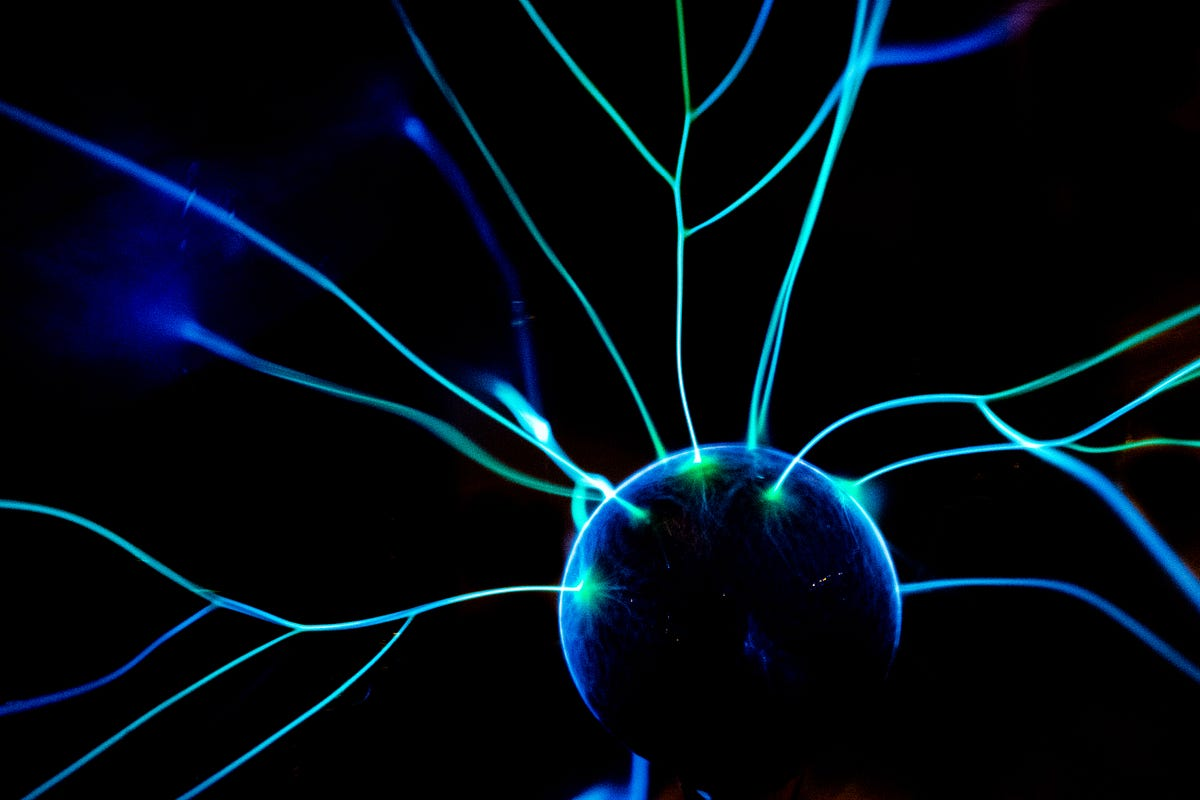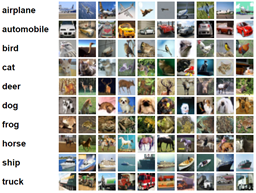# Integration of Dimension Reduction Methods and Neural NetworkBuilding a deep network using original digital images requires learning many parameters which may reduce the accuracy rates. The images can be compressed by using dimension reduction methods and extracted reduced features can be feeding into a deep network for classification. Hence, in the training phase of the network, the number of parameters will be decreased. Principal Component Analysis is a well-known dimension reduction technique that leverages the orthogonal linear transformation of the original data.

Building a deep network using original digital images requires learning many parameters which may reduce the accuracy rates. The images can be compressed by using dimension reduction methods and extracted reduced features can be feeding into a deep network for classification. Hence, in the training phase of the network, the number of parameters will be decreased. Principal Component Analysis is a well-known dimension reduction technique that leverages the orthogonal linear transformation of the original data. In this article, we demonstrate a neural network-based framework, named Fusion-Net, which implements PCA on an image dataset (CIFAR-10), and then a neural network applies to the extract principal components. We also implemented logistic regression on the reduced dataset. Finally, we compare between results of using original features and reduced features. The experimental results show that Fusion-Net outperformed other methods.

In this article, we apply a neural network framework named Fusion-Net for image classification. The implementation follows two steps:

1. We implement the dimension reduction technique (PCA) to the original dataset to extract principal components
2. The extracted components are then used as input to the ML classifiers like Neural Network, Logistic regression, etc.

Dataset specification

We performed experiments on an image dataset, named CIFAR. The CIFAR data set is a well-known image data for multi-class classification of 10 classes of images which are collected by Alex Krizhevsky, Vinod Nair, and Geoffrey Hinton . The images in the dataset have 10 different classes of objects: airplane, automobile, bird, cat, deer, dog, frog, horse, ship, and truck.

Fig. 1 illustrates some of the sample images with class labels of the images. In this article, we used the CIFAR-10 dataset consists of 60,000 images which are a subset of 80 million tiny images dataset (CIFAR). Each of the images in the dataset is a color image (containing 3 channels) with dimensions. CIFAR-10 dataset is a balanced data where each of the classes contains 6,000 images. We split the dataset into 50,000 training images and 10,000 test images preserving the proportion of classes.## Satellite image classification with a convolutional neural network.

My latest project at Flatiron was to use neural networks to classify satellite image tiles. I chose to use a convolutional neural network (CNN).

## Feature pyramid network for image classification

Feature pyramid network for image classification. Here, I aim to introduce a new architecture based on FPN to improve classification accuracy. This architecture is proposed in my paper.

## Preprocessing your images for machine learning (image recognition)

During my studies at JKU there was a task for preprocessing images for a machine learning project. It is necessary to clean the raw images…

## Hire Machine Learning Developers in India

We supply you with world class machine learning experts / ML Developers with years of domain experience who can add more value to your business.

## Learn Machine Learning with Python (Part 3) | Machine Learning with Neural Networks

Learn Machine Learning with Python using neural networks with this machine learning beginners course. In this tutorial we will look at taking an existing sol...